# Linear Learning Models¶

In :
from ipypublish import nb_setup


## Logistic Regression¶

The subject of this chapter are Linear Neural models used for classification, which also go by the name Logistic Regression. These models serve as the building block for more sophisticated DLN classification models, all of whom use a Logistic Regression layer for their final stage. We proceed in two stages, we start with the case $K=2$ for classification into two categories, followed by the general case of $K$ categories.

In :
#LR10
nb_setup.images_hconcat(["DL_images/LR10.png"], width=600)

Out: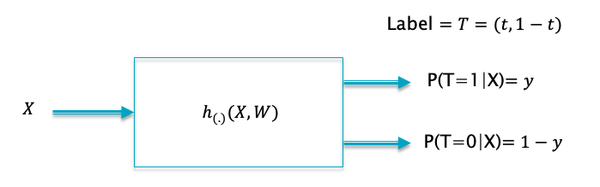In Figure LR10 we reproduce the classification model from the previous chapter, specialized for the case $K=2$. The model processes the input $X$ and produces a probability distribution for the two output values. Not that only a single output $P(T=1|X) = y$ is needed, since the other output is always $P(T=0|X)=1-y$. We now propose our first model for the function $h$, which is shown in Figure LR2.

In :
#LR2
nb_setup.images_hconcat(["DL_images/LR2.png"], width=600)

Out: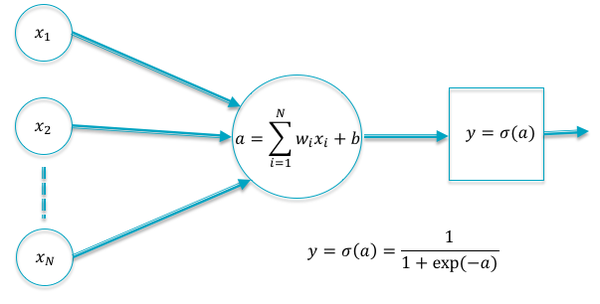## Sigmoid Function¶

Using the notation from the previous chapter, the functional form of the simplest linear model with single node in the Output Layer (corresponding to $K=2$) is given by

In this equation $(w_1,...,w_N)$ are called weights and $b$ is known as the bias (see Figure LR2)). As the reader can readily see, $a$ can not function as the output of a classification system, since we cannot ensure that $0 \le a\le 1$. In order to satisfy this requirement we add a function called the sigmoid to the system (see Figure sigmoid). The sigmoid function is also referred to as the “squashing function” since it maps the entire real axis into the finite interval $[0, 1]$. The resulting output $y$ satisfies the condition $0\le y\le 1$ and hence is a suitable output for the Logistic Regression system.

$$y = \sigma(a) = \frac{1}{1+\exp(-a)}$$
In :
#sigmoid
nb_setup.images_hconcat(["DL_images/sigmoid.png"], width=600)

Out: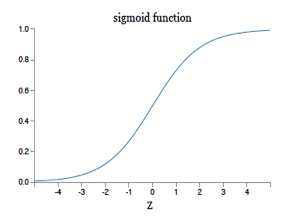We can do classification using this model by using the following criteria: If $y\ge 0.5$, then the model predicts that input to be in class 1, or else in class 2. Note that the condition $y\ge 0.5$ is equivalent to the condition $$a = \sum_{i=1}^N w_i x_i + b \ge 0$$ so that input points on one side of the hyperplane $\sum_{i=1}^N w_i x_i + b$ are categorized in class 1, and those on the other side are categorized in class 2. This leads to the question: Why not use $a$ to do the classification, thus avoidng the extra step of computing $y$. If we use the criteria $y\ge 0.5$ to do the classification, then indeed there is no difference in whether we choose to use $y$ or $a$. However if we change the classification criteria to the following: Compute $y$ and use it to generate a Random Number such that the probability of the input being in class 1 is $y$, and similarly for class 2. With this criteria, there is a finite chance that the predicted class is $2$, even though $y\ge 0.5$ (especially if $y$ is close to $0.5$). This means that input points that are close to the hyperplane $a = \sum_{i=1}^N w_i x_i + b$ have an equal chance of being classified in either Class 1 or Class 2. This distinction is important, since it enables the Logistic Regression model to use probabilistic inference and do classification even in cases in which the two classes are not strictly linearly separated. The interpretation of the Linear Model as a hyperplane also explains the need for the bias parameter $b$, since in the absence of $b$, all hyperplanes would be constrained to pass through the origin, which obviously limits their modeling power.

## Linear Separator¶

In :
#linearSeparator
nb_setup.images_hconcat(["DL_images/linearSeparator.png"], width=600)

Out: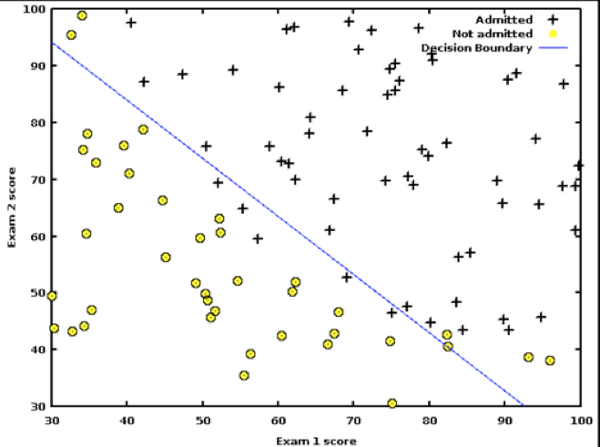Figure linearSeparator shows the results of applying Logistic Regression in the two dimensional case to a test dataset. As we noted earlier, point that are close to the linear separator may get classified in either of the two classes due to the statistical nature of the algorithm.

Now that we have obtained the structural form for our classification system for the special case $K=2$, the next step is to estimate the model parameters $b, w_i, i=1,...,N$ from the training data. From the discussion in the prior chapter, this can be done by choosing the parameters so that they minimize the Cross Entropy Loss Function. Given that $(X{(m)},T{(m)}),m=1,...,M$ is the training sequence, the Loss Function is defined as:

$$L = -{1\over M}\sum_{m=1}^M [t{(m)} \log y{(m)} + (1-t{(m)}) \log(1-y{(m)})]$$

where the output $y{(m)}$ in response to the $m^{th}$ input vector $X{(m)}$ is given by

$$y{(m)} = \sigma(a{(m)})\ \ where\ \ a{(m)} = \left(\sum_{i=1}^N w_i x_i{(m)} + b \right), \ \ 1\le m\le M$$

Even for this simple model there is no closed form solution for determining the values of $(W,b)$ that minimize $L$, so we resort to the use of the Gradient Descent algorithm:

$$w_i \leftarrow w_i - \eta \frac{\partial L}{\partial w_i},\ \ 1\le i\le N\ and\ b\leftarrow b - \eta \frac{\partial L}{\partial b}$$

where $\eta$ is a constant called the Learning Rate. There are various ways in which the iteration shown above can be improved and is discussed in detail in Chapter GradientDescentTechniques. In order to proceed we need to compute the gradients $\frac{\partial L}{\partial w_i}$ and $\frac{\partial L}{\partial b}$.

The Loss Function $\mathcal L$ for a single sample of the training dataset is given by:

$$\mathcal L = -[t\log y + (1-t)\log (1-y)]$$

Using the Chain Rule of Derivatives,

$$\frac{\partial \mathcal L}{\partial w_i} = \frac{\partial \mathcal L}{\partial y}\frac{\partial y}{\partial a}\frac{\partial a}{\partial w_i}$$

Note that

$$\frac{\partial \mathcal L}{\partial y} = \frac{y-t}{y(1-y)},\ \ \frac{\partial y}{\partial a} = y(1-y)\ \ and\ \ \frac{\partial a}{\partial w_i} = x_i$$

which results in

$$\frac{\partial \mathcal L}{\partial w_i} = x_i(y-t),\ 1\le i\le N$$

We can now write down the expression for the derivative of the Loss Function $L$ for the entire training dataset:

\begin{equation} \frac{\partial L}{\partial w_i} = {1\over M}\sum_{m=1}^M x_i(m)(y{(m)}-t{(m)}),\ 1\le i\le N \quad \quad \mbox{(**dLdW**)} \end{equation}

In :
#LR11
nb_setup.images_hconcat(["DL_images/LR11.png"], width=600)

Out: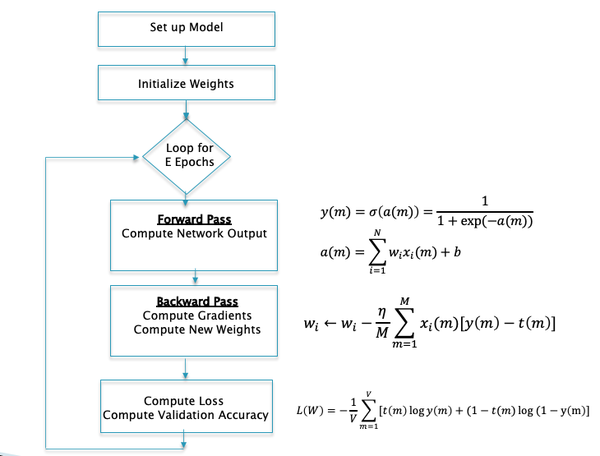Using equation dLdW, the following iterative algorithm can be used to train the model:

1. Initialize all the weights $w_i, 1\le i\le N$ and the bias term $b$ to zero.

2. In response to the training sequence $X{(m)}, 1\le m\le M$, compute the model predictions $y(m), 1\le m\le M$ (using the latest values of the parameters $(W,b)$): $$y(m) = \sigma\left(\sum_{i=1}^N w_i x_i{(m)} + b \right), \ \ 1\le m\le M$$

3. Change the model parameters according to the formulae

\begin{equation} w_i \leftarrow w_i - {\eta\over M} \sum_{m=1}^M x_i(m)(y{(m)}-t{(m)}),\ 1\le i\le N \quad \quad \mbox{(**L1**)} \end{equation}$$b \leftarrow b - {\eta\over M} \sum_{m=1}^M (y{(m)}-t{(m)})$$
1. Compute the Loss Function $L$ over the Validation Dataset given by $$L = -{1\over V}\sum_{m=1}^V t{(m)} \ln y{(m)} + (1-t{(m)} \ln(1-y{(m)})$$ where $V$ is the number of samples in the Validation Dataset. If $L$ drops below some threshold, then stop. Otherwise go back to Step 2.

The Gradient Descent Algorithm is our first encounter with training or learning algorithms in this monograph. The mathematical setup and general procedure that we used in this algorithm holds true for more complex models. Step 2 of the algorithm in which the output of the model is computed is known as the Forward Pass. Step 3 of the model in which the gradients are computed and then used to update the model parameters, is known as the Backward Pass. This model (and all other DLN models) proceeds by repeated cycles of Forward and Backward Passes, until the stopping condition is satisfied. This is also illustrated in Figure LR11. All DLN training algorithms share this structure of alternating Forward and Backward passes, the only difference being that the calculations become more complex in the Forward Pass and the Backward Pass is implemented using the Backprop algorithm.

An alternative to using the Loss Function as a stopping criteria, is to use a performance measure called Accuracy. This is defined as the percentage of correct predictions on the Validation Dataset, so that, the Accuracy $A$ is given by $$A = \frac{ \sum_{m=1}^V 1_{y(m)=t(m)}} {V}$$ If the value of $A$ exceeds some threshold, terminate the algorithm.

We can get some intuition into the workings of this training algorithm as follows: Consider a training example in which the label $t=1$. From Equation L1 it follows that the training iteration is given by $$w_i \leftarrow w_i - {\eta\over M} \sum_{m=1}^M x_i(m)(y(m)-1)$$ Since $y-1 \le 0$, it follows that all the weights $w_i$ increase during this iteration of the training loop. This would cause the the value of $y$ to increase for the input $X$. This makes intuitive sense, since we would want the probability of $X$ being classified in Category 1 to increase as a result of this training sample.

Conversely, if the label for the input $X$ was $t=0$, then the training iteration becomes: $$w_i \leftarrow w_i - {\eta\over M} \sum_{m=1}^M x_i(m) y(m)$$ This expression shows that the iteration results in a decrease of model weights, so that next time the same input is presented, the out $y$ of the model will be smaller. As expected this implies that the probability of $X$ being classified in the Category 1 decreases as a result of this training sample.

In ML algorithms, an epoch is defined as the time required to do a single pass through all the elements in the training dataset. In the algorithm described above, note that model parameters are updated only once per epoch. In practice, this considerably slows down the speed of convergence, especially for large training datasets. We now present a modification to the algorithm, called Stochastic Gradient Descent (SGD), which updates the parameters more frequently, indeed after processing each element in the training dataset. The key to SGD is the observation that the parameter update equation can be written as:

\begin{equation} w_i \leftarrow w_i - \eta x_i(m)(y{(m)}-t{(m)}),\ 1\le i\le N \quad \quad \mbox{(**L2**)} \end{equation}

Hence for input vector $X(m)$, we compute the corresponding output $y(m)$, and then use the above equation to immediately change the model parameters, and then repeat the process with the next input. This considerably speeds up the algorithm, and thus the learning process. Effectively by doing this, we are using noisy estimates of the gradient to do the iteration, which causes the convergence to be not as smooth as with Gradient Descent (see Figure SGD). However it has been observed that the noise introduced to SGD also has the benefit of helping the algorithm avoid getting stuck in non-optimal minima, as well as escape from saddle point quickly.

In :
#SGD
nb_setup.images_hconcat(["DL_images/SGD.png"], width=600)

Out: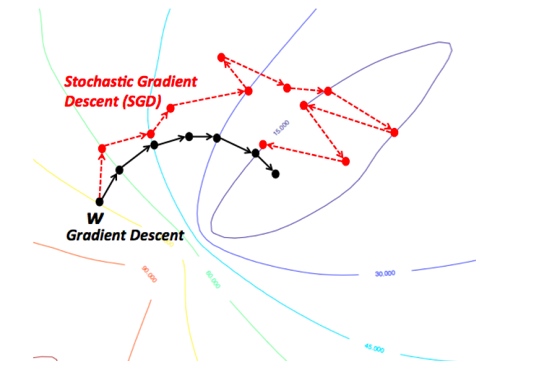## Stochastic Gradient Descent Algorithm (SGD)¶

1. Initialize all the weights $w_i, 1\le i\le N$ and the bias term $b$ to zero.

2. For $m = 1,...,M$ repeat the following steps (a) - (c):

(a) For the input vector $X{(m)}$, compute the model prediction $y(m)$ given by $$y(m) = \sigma\left(\sum_{i=1}^N w_i x_i{(m)} + b \right)$$

(b) Change the model weights according to the formula

$$w_i \leftarrow w_i - \eta x_i(m)(y{(m)}-t{(m)}),\ 1\le i\le N$$$$b \leftarrow b - \eta (y{(m)}-t{(m)})$$
(c) Increment $m$ by one and go back to step $(a)$.


1. Compute the Loss Function $L$ over the Validation Dataset given by $$L = -{1\over V}\sum_{m=1}^V t{(m)} \log y{(m)} + (1-t{(m)} \log(1-y{(m)})$$ If $L$ has dropped below some threshold, then stop. Otherwise go back to Step 2.

Note that in the SGD algorithm we update the parameter values after processing each element in the training dataset, but we check the stopping condition only once per epoch.

## Batch Stochastic Gradient Descent Algorithm (B-SGD)¶

There is a third way of solving the optmization problem, which is midway between the two extremes of Gradient Descent and Stochastic Gradient Descent, and is known as Batch Stochastic Gradient Descent (B-SGD). This is based on the following parameter update rule: $$w_j \leftarrow w_j - {\eta\over B} \sum_{b=1}^B x_i(b)(y{(b)}-t{(b)})$$ This algorithm works by dividing up the training dataset into batches of size $B$, and then updates the parameters only once per batch. By varying the batch size, we can trade-off the smoothness of the convergence algorithm with the speed of convergence.

1. Initialize all the weights $w_i, 1\le i\le N$ and the bias term $b$ to zero.
2. For $r = 0,...,{M\over B}-1$ repeat the following steps (a) - (c):

(a) For the training inputs $X_i(m), rB\le i\le (r+1)B$, compute the model predictions $y(m)$ given by $$y(m) = \sigma\left(\sum_{i=1}^N w_i x_i{(m)} + b \right)$$ (b) Change the model weights according to the formula $$w_j \leftarrow w_j - {\eta\over B} \sum_{m=rB}^{(r+1)B} x_i(m)(y{(m)}-t{(m)}),\ 1\le j\le N$$ $$b \leftarrow b - {\eta\over B} \sum_{m=rB}^{(r+1)B}(y{(m)}-t{(m)})$$

(c) Increment $r$ by one and go back to step $(a)$.

3. Compute the Loss Function $L$ over the Validation Dataset given by $$L = -{1\over V}\sum_{m=1}^V t{(m)} \ln y{(m)} + (1-t{(m)} \ln(1-y{(m)})$$ If $L$ has dropped below some threshold, then stop. Otherwise go back to Step 2.

In :
#GDComp
nb_setup.images_hconcat(["DL_images/GDComp.png"], width=600)

Out: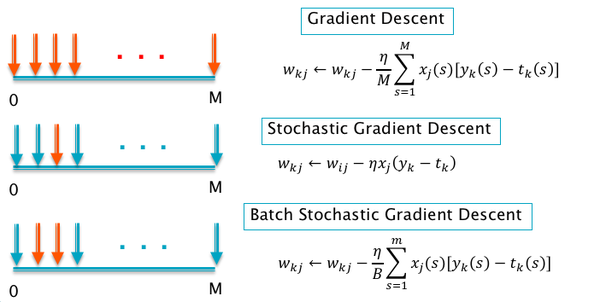Figure GDComp gives a summary of the three Gradient Descent algorithms types, with the red arrows representing the number of input samples that are used in the computation of a single update of the model parameters.

## Multiclass Logistic Regression¶

In :
#multiclassLogit
nb_setup.images_hconcat(["DL_images/multiclassLogit.png"], width=600)

Out: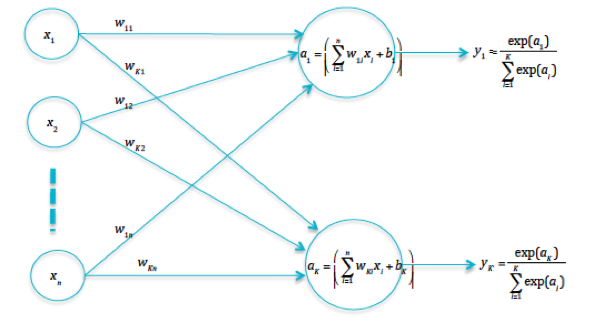If the input can belong to one of $K$ categories, then the Logistic Regression model can be expanded to accommodate this, as shown in Figure multiclassLogit. Define the quantities $a_k$ by

$$a_k = \sum_{i=1}^N w_{ki} x_i + b_k, \quad 1 \leq k \leq K$$

These quantities are referred to as logits in Machine Learning literature, and are defined as the outputs of the final layer of neurons. They are also sometimes referred to as "Scoring Functions", since a higher score for a class means that it stands a greater chance of being the selected category.

We need to convert these logits into output probabilities $y_k$. Note that the sigmoid function cannot be used for this, since in that case the condition $\sum_{k=1}^K y_k = 1$ is not guaranteed to be satisfied. Instead the probabilities are generated using the following equation:

$$y_k = \frac{\exp(a_k)}{\sum_{i=1}^K \exp(a_i)}, \quad 1 \leq k \leq K$$

which is called the Normalized Exponential or Softmax function and is a multiclass generalization of the sigmoid. Indeed for the case $K = 2$ it is easy to show that softmax and the sigmoid result in identical outputs.

Using the results from the previous chapter on Supervised Learning, the Loss Function for this model can be written as

$$L = -{1\over M}\sum_{m=1}^M \sum_{k=1}^K t_k(m)\log y_k(m) = {1\over M}\sum_{m=1}^M \mathcal L(m)$$

where

$$\mathcal L(m) = -\sum_{k=1}^K t_k(m)\log y_k(m)$$

In these equation, the labels $t_k$ are defined such that if the label corresponds to the $q^{th}$ category, then $t_q = 1$ and $t_k = 0, k\ne q$. In order to train the model using Gradient Descent, we need to compute $\frac{\partial \mathcal L}{\partial w_{ki}}$ and $\frac{\partial \mathcal L}{\partial b_{k}}$. Using the Chain Rule of derivatives

$$\frac{\partial \mathcal L}{\partial w_{ki}} = \frac{\partial \mathcal L}{\partial a_{k}}\frac{\partial a_k}{\partial w_{ki}}$$

Note that $\frac{\partial a_k}{\partial w_{ki}} = x_i$ while $\frac{\partial \mathcal L}{\partial a_{k}}$ can be written as

$$\frac{\partial \mathcal L}{\partial a_{k}} = \sum_{j=1}^K \frac{\partial \mathcal L}{\partial y_{j}} \frac{\partial y_j}{\partial a_{k}}$$

In order to justify this equation, note that the logit $a_k$ influences the Loss Function through all of the outputs $y_j, 1\le j\le K$, due to the form of the softmax equation. Note that

$$\frac{\partial \mathcal L}{\partial y_{j}} = -\frac{t_j}{y_j}$$

while it is not difficult to show that

$$\frac{\partial y_j}{\partial a_{j}} = y_j(1-y_j)$$

and

$$\frac{\partial y_j}{\partial a_{k}} = -y_jy_k\ \ for\ \ j\ne k$$

It follows that

$$\frac{\partial \mathcal L}{\partial a_{k}} = -\frac{t_k}{y_k} y_k(1-y_k) - \sum_{j\ne k}\frac{t_k}{y_j}(-y_jy_k) = -(t_k - t_ky_k-\sum_{j\ne k}t_jy_k) = -(t_k-\sum_{j=1}^K t_jy_k) = y_k-t_k$$

Putting all these equations together, it follows that

$$\frac{\partial \mathcal L}{\partial w_{ki}} = x_i(y_k - t_k)$$

Similarly it can be shown that

$$\frac{\partial \mathcal L}{\partial b_{k}} = (y_k - t_k)$$

Using these expressions, we end up with the following Gradient Descent Training Algorithm for Logistic Regression systems with $K$ output categories:

In :
#LR12
nb_setup.images_hconcat(["DL_images/LR12.png"], width=600)

Out: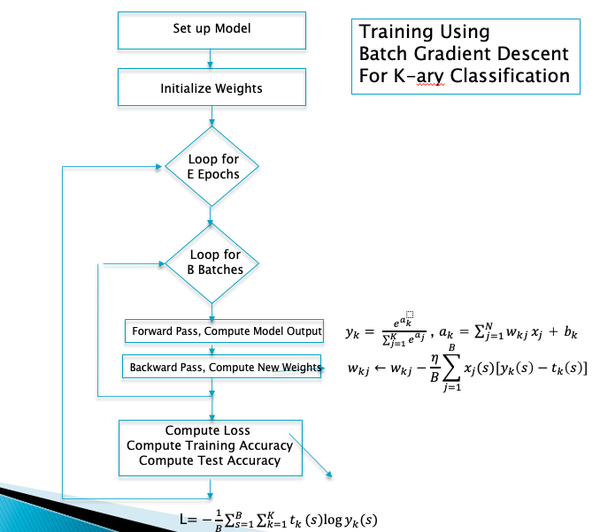1. Initialize all the weights $w_{ki}, 1\le k\le K, 1\le i\le N$ and the bias terms $b_k, 1\le k\le K$ to zero.

2. Loop through (a) and (b) for $M\over B$ batches:

a. Forward Pass: In response to the training sequence $X{(m)}, 1\le m\le M$, compute the model predictions $y_k(m), 1\le k\le K, 1\le m\le B$ (using the latest values of the parameters $(W,b)$):

$$y_k(m) = {{\exp{a_k(m)}}\over{\sum_{i=1}^K \exp{a_i(m)}}},\ \ where\ \ a_k(m) = \sum_{i=1}^N w_{ki} x_i{(m)} + b_k,\ \ 1\le k\le K,\ \ 1\le m\le B$$
b. Backward Pass: Change the model parameters according to the formulae


$$w_{ki} \leftarrow w_{ki} - {\eta\over B} \sum_{m=1}^B x_i(m)(y_k{(m)}-t_k{(m)}),\ 1\le k\le K,\ 1\le i\le N$$$$b_k \leftarrow b_k - {\eta\over B} \sum_{m=1}^B (y_k{(m)}-t_k{(m)}), \ \ 1\le k\le K$$
1. Compute the Loss Function $L$ over the Validation Dataset given by
$$L = -{1\over V}\sum_{m=1}^V\sum_{k=1}^K t_k{(m)} \log y_k{(m)}$$

where $V$ is the number of samples in the Validation Dataset. If $L$ drops below some threshold, then stop. Otherwise go back to Step 2.

Just as for the case $K=2$, we can verify that the weight update equations in this algorithm cause the output probabilities to change in the right way, as follows: Consider the case that the input $X$ is such that the label corresponds to the $q^{th}$ category so that $t_q = 1$ and $t_k = 0, k\ne q$. Then the weight updates can be written as

$$w_{qi} \leftarrow w_{qi} - {\eta\over M} \sum_{m=1}^M x_i(m)(y_q{(m)}-1),\ \ 1\le i\le N$$

and

$$w_{ki} \leftarrow w_{ki} - {\eta\over M} \sum_{m=1}^M x_i(m)y_k{(m)},\ \ k\ne q,\ 1\le i\le N$$

From these equations it is clear the weights that are incident on the $q^{th}$ output increase, while all the other weights decrease. Hence it follows that the next time the same input is sent into the model, the value of the probability $y_q$ will be higher and the other $y_k, k\ne q$ will be smaller.

## Image Classification Using Linear Models¶

In :
#LC1
nb_setup.images_hconcat(["DL_images/LC1.png"], width=600)

Out: